Investigation on Keynesian Monetary Fluctuations | OMICS International

# Investigation on Keynesian Monetary Fluctuations

Byung Woo K*

Korea National University of Transportation (KNUT), Korea

*Corresponding Author:
ByungWoo K
Korea National University
of Transportation (KNUT)
72 DaehangNo
Chung Ju Korea -380-702
Tel: 010-6735-7401
E-mail: [email protected] cjnu.ac.kr

Received date: July 25, 2015 Accepted date: August 17, 2015 Published date: August 24, 2015

Citation: Byung Woo K (2015) Investigation on Keynesian Monetary Fluctuations. Bus Eco J 6:174. doi:10.4172/2151-6219.1000174

Copyright: © 2015 Byung Woo K. This is an open-access article distributed under the terms of the Creative Commons Attribution License, which permits unrestricted use, distribution, and reproduction in any medium, provided the original author and source are credited.

Visit for more related articles at Business and Economics Journal

#### Abstract

We examined money-output regression (St. Louis equation) considering econometric problem, macroeconomic theory, and policy implications. Our results reveal that increases in money affects real variables like output over a few quarters. These results ascertain Keynesian macroeconomic view that price-wage rigidity cause output to respond to nominal shocks like shifts in money demand. Out derivation adds contribution to the original empirical results with regard to; endogeneity of money, lags from monetary shocks on output, and serial correlation in output disturbances (persistence), and fundamental equilibrium relationship (co-integration), etc.

#### Keywords

St. Louis equation; Real business-Keynesian theories; Structural VAR; IS-MP model

JEL Classification: E32, O30

#### Introduction

Keynesian economic theory assumes that there is rigidity in price and wage . Hence, we need to know aggregate supply to determine output level. In this model, monetary disturbances have real effects on employment and output. In contrast, real-business cycle models argue that purely monetary disturbances have no real effects . One of the methods to determine which is right is famous St. Louis equation (Anderson and Jordan, 1968).

Friedman and Schwartz  analyzed the sources of movements in the money and concluded that financial development is main source of fluctuations of money rather than real developments. Blanchard and Quah  show that aggregate demand shock in money supply equation has transitory effect on output in contrast to the fact that supply shock has permanent effect. Cecchtti and Rich (2001) presented a structural VAR that provides two-equation model of inflation and output. They calculated the sacrifice ratio, which is the amount of decrease in GDP for disinflationary policy. This was used to study the long-run real effects of monetary policy.

In classical economists, money plays only the role of determining the price level. It cannot affect real variables like output. This phenomenon is called as the neutrality of money. Money determines aggregate demand, but not production, since output (aggregate supply) is fixed at the full employment level. New classical economists including real-business fluctuation theory also has the same view. In contrast, Keynesian economists argue that money affects output through transmission mechanism.

In this study, we investigate these issues with regard to output regression method. The following questions should be answered to determine the existence of real effects of money stock from St. Louis equation estimation. This equation is important in that it is important to know the actual effect of monetary policy in boosting the economy. Since 2008 global financial crisis, worldwide recession caused the government to think on appropriate instrument for stabilizing the national economy.

First, does causation run from money to output? Full understanding for monetary policy requires the path through which output and money affect each other including transmission mechanism.

Second, what are the determinants of monetary policy? Policy may be preemptive (discretionary) or of rule.

Third, we should consider the shift of money demand due to deregulation and financial innovation. In 1980s, financial innovation affected money demand with regard to transaction costs, etc.

Fourth, are there any other sophisticated statistical methods than ordinary regression? Recently, various econometric models like error correction, GARCH, and polynomial distributed lagged model, etc., have been developed rapidly.

Sixth, we solve several econometric problems related with moneyoutput regression like St. Louis equation. These are problems of autocorrelation, endogeneity, omitted variables and reverse causation (Cooley and LeRoy, 1985).

We compare macroeconomic structures of the economies through comparison of estimation results. Our research is mainly based on graduate textbook level like Romer . Figure 1 shows the growth of money and output in graph. Money stock seems to be procyclical and leading variable. It is well known that M1 is procyclical and leading in Korea. Its volatility is about three times higher than that of GDP. M1 includes cash, demand deposit, MMDA (money market deposit account) and MMF (money market fund).

Recently, Federal Reserve performed QE (quantitative easing) policy for providing liquidity for markets and its tapering. In general, short-run stabilization of macroeconomy in most countries is performed by monetary policy rather than fiscal policy. We summarize the aspects of monetary policy in brief. Fisher equation, which shows the relation between interest rate and inflation, is expressed as follows; R=r+π(e).

Inflation coming from the increase of money stock in short-run is called liquidity effect. It lowers nominal interest rates in negative

direction. But, in long run, the change in money stock affects nominal rates one-for-one; Fisher effect. We assume that real interest rate is unaffected by inflation. But, if we allow the real interest rate to change, then liquidity effects also imply the real rates being able to decrease.

Monetarist Friedman argues that the increase in money stock causes the nominal income to increase responsively. We want to know how much the real income to increase in response to monetary shocks. Neutrality of money asserts that one-time increase in nominal money stock doesn’t affect the real sector variables.

Monetary-business cycle theory presents the mechanism through which monetary shock affects output. It emphasizes the misperception of workers, which leads to the illusion between real and nominal wages.

#### Econometric Model of Monetary Policy

Interest rates, output and monetary policy

First, we construct IS-MP diagram. 

Y=C(Y-T)+I(r)+G

M/P=L(r+πe, Y)

In this simple model, money is considered as high-powered money, that is, the sum of currency and reserves. We can analyze the mechanism through which money affects the interest rate and output.

The second equation shows the equilibrium condition in the money (financial) market. It expresses inflation as a function of the money stock.

P=M/L(R, Y)

If there is permanent increase in money growth, the price level grows at the same rate. Although real interest rate is constant in shortrun, nominal rate shows the path changing after some pattern. Main determinant of inflation is money growth. The fluctuation of nominal interest rate comes from expected inflation component. Short-term variation of real rate is limited. Meanwhile, if price is incompletely flexible, effects on nominal rate is important.

In this study, we mainly study the paths of output and nominal interest rate after IS-MP macroeconomic model. We follow the response path of output and nominal rate due to increase in money.

Distributed lags (DL); policy analysis

We use structural VAR for estimating the effects of money on GDP. This model overcomes the disadvantage of reduced VAR, among which the depletion of theoretical component is most serious. So many economic researches are based on this model .

First, we examine the relationships between monetary changes and business cycle. The following results show that monetary disturbances implies future boom in the economy.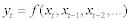where x: M1, y: real GDP

Data used in regression are money and output in the U.S. during 1947-2007.

A natural test of real business vs. Keynesian theories was performed by Anderson and Jordan (1968). This regression of output growth on money growth is known as St. Louis equation.

This specification is called finite distributed lags (FDLs) in econometrics. The sum of the coefficients on the current and two lagged values of money growth are 0.16, with a standard error of 0.07. It means that 1% increase in the money stock is associated with (1/6) increase in output over the two quarters later.

It is important to note that in the presence of autocorrelation, OLS estimator is no longer best. It means that hypothesis testing can be misleading in St. Louis equation.

Second degree PDL takes the form: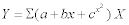We estimated delay multipliers and drawn their graph in Figure 2. It seems to coincide with the estimates for DL model (Tables 1 and 2).

OLS
Null Hypothesis : The following coefficients are zero (non causality)
∆M1 Lag(s) 0 to 2
F(3,196) 2.0 with significance level 0.11
Summary of linear combination of coefficients(interim multiplier)
∆M1 Lag(s) 0 to 2
Value 0.15
Standard error 0.07
Durbin-Watson statistic 1.3
t-statistic 2.2
Signif level 0.02**
GLS considering autocorrelation (Cochrane-Orcutt procedure)
Null Hypothesis : The following coefficients are zero (non causality)
M1DIFF Lag(s) 0 to 2
F(3,194) 3.56 with significance level 0.01
Summary of linear combination of coefficients(interim multiplier)
M1DIFF Lag(s) 0 to 2
Value 0.22
Standard error 0.09
t-statistic 2.31
Signif level 0.02**

Table 1: Causality in finite distributed lags model with and without autocorrelation: St. Louis Equation.

 Exogeneity of nominal variables (M2 P) Log determinants: 20.8 21.4 Chi-squared(24)=86.6 with significance level 0.00 Null hypothesis : The following coefficients are zero ∆M2 Lag 0 to 12 F(13,126)=1.83 with significance level 0.04

Table 2: Reduced form VAR model; Exogeneity.

We use VAR for defining Granger no causality (strong exogeneity) of money, when lagged values of M do not provide information about the conditional mean of Y.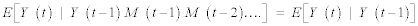The test for this no causality is performed for testing zero coefficients using F-test.

Vector autoregression (VAR); policy analysis

We use structural VAR for estimating the effects of money on GDP. This model overcomes the

Second, Let’s see the standard form of reduced VAR.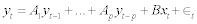The structural error is obtained from linear transformation of reduced form error. If we transform the notation of the structural error into u and reduced form error into e:

Aet=But

Main advantage of structural VAR is that we can impose so many restrictions corresponding to theory (for example, supply side does not affect demand side in macroeconomy). The main idea is that we can overcome reduced form VAR devoided of structural or theoretical component. Economists made assumptions about the conduct of policy that allow the estimates of VAR to be mapped into policy’s impact. These structural VARs were pioneered by Sims , Bernanke etc. Cecchtti and Rich (2001) presented a structural VAR for analyzing disinflationary policies of the Fed on output.

We can see this problem from different side: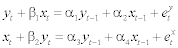structural VAR, e is not observed, but should be identified from observed reduced form error (or residuals).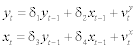: reduced form VAR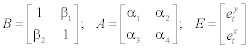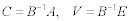Identification condition is: B=I

We use this model for estimating impulse response function of GDP for the shock of the change in monetary aggregate. We used monthly data set from BEA website for the U.S. economy. Variables include real GDP(Y), consumption(C), physical investment (I), government purchases (G), money stock (M1) and inflation rate (P) from 1948 to 2007 (Figure 3).

We can approach more wide issues at this stage. First, we can construct AD-AS model with structural VARs as . They do not consider explicitly the effects on output of money growth. Second, money-inflation relation is also important. We can decompose the effects of monetary policy into on output and the price level separately. Cecchtti and Rich (2001) presented a structural VAR that presents twoequation model of inflation and output. In structural VAR, the equation system is written by: [Δ (output), Δ (inflation)] the components of the disturbance (innovation) are identified as shocks to AS (aggregate supply) and AD, respectively. To calculate the impact of structural shocks, they use the VMA (vector moving average) form. Blanchard and Fischer  showed that aggregate demand shocks caused by changes in the money in the face of incomplete adjustment of wages and prices have transitory effects on output. Relative importance of aggregate demand and supply shocks are main theme of macroeconomics.

Aggregate demand and supply disturbances .

Macroeconomic model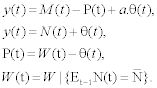Y: output M: money P: price level θ: productivity

N: employment W: nominal wage

#### Sophisticated Statistical Evidence

In this study, we investigate the following issues:

• First, does causation run from money to output?

We performed exogeneity test using F and t tests. The lagged variables of money are significant at 5% level. The hypothesis of endogeneity is rejected at high level of significance. Our causality tests show that causation run bilaterally. King and Plosser  argue that when firms increase their production, they may increase money holdings. Measures of money, such as M1, are set by Federal Reserves. We can think that they increase high-powered money when there occurs economic boom. In addition, increase in money stock boosts economy through transmission mechanism. There are two different ways by which money affects aggregate demand; Keynesian vs. Monetarist. We need to know the accurate lags through which money and output affect each other. Our exogeneity tests show that the causation between money and output runs bilaterally. Romer  argues that there is possibility of causation from output to money, but we got empirical evidence for this argument (Table 3).

 Exogeneity of real variables (GDP C I G) Chi-squared(24)=62.2 with significance level 0.00** Exogeneity of nominal variables (P M2) Chi-squared(6)=23.4 with significance level 0.00**

Table 3: Exogeneity tests of real vs. nominal variables.

• Second, what are the determinants of monetary policy?

We can choose measures of monetary policy other than M1. The choice is Federal Funds Rate. Finite distributed lags model shows that interim multiplier has significant negative value. In addition to output, we can regard the determinants of policy as inflation. Karenken and Solow (1963) argue that we can observe fluctuations in money and stable output, if Federal Reserve’s efforts to stabilize the economy are successful. Kuttner  observed that Federal Reserve’s open market operations are associated with nominal interest rates. FRB’s target is associated with actual funds rate, which shows strong evidence of causality from monetary policy to short-term interest rates. We found other factor, interest rate rather than money that influences aggregate output (Table 4 and Figure 4).

Summary of linear combination of coefficients
FFED Lag(s) 0 to 12
Value -4.23e-04
Standard error 2.42e-04
t-statistic -1.75
Signif level 0.08*

Table 4: Effects of federal funds rate on output.

Note) 1 Cheong et al. (2015) Macroeconomics (Korean)
2 Red lines are for the US.
Figure 4: Trend of federal funds rate.

• Third, we should consider the shift of money demand stemming from deregulation and financial innovation.

According to Stock and Watson (2004), consumption smoothing due to financial innovation contributed to the Great Moderation. This affects interest rate, prices and output through shift of money demand. The great moderation is said to occur in the midtime of 1980s. Our Chow test shows that there is no equivalence of equations over two periods. This means that there have been a series of large shifts in the demand for money over the past three decades. Keynesian theory asserts that if there occurs positive money demand shocks, there is negative relation between money and output. That is, when Fed does not intervene in money market, increase in money stock and high interest rate coexists. Korean case of money demand shift is summarized by Kim .

• Fourth, due to money demand shifts, estimation is sensitive to sample period, and measure of money.

We examined this argument by estimating equations through different sample periods and M1, M2, respectively. We know that changing measure of money from M1 to M2 causes the estimates in St. Louis equation to vary in significant amount.

• Fifth, is there any other sophisticated statistical method than ordinary regression?

Original St. Louis equation suffered from omitted variables, money-demand shifts and causation, etc. (Cooley and LeRoy, 1985). Money-output regression may suffer from endogeneity problem. In this case, OLS estimators are inconsistent. They do not converge to the true value even in the large sample.

Proper instrument variable should be correlated with money growth and not with the other factors that affect real output. We selected physical investment and Federal Funds Rate. Two-stage least squares estimation reduces to larger estimate than that of OLS. In finite distributed lags (FDLs) the sum of the coefficients on the current and two lagged values (over two months) of money growth is 0.16. It means that 1% increase in the money stock is associated with (1/6) increase in output over the two months later in contrast to 0.22 in GLS method. It is important to note that in the presence of autocorrelation, OLS estimator is no longer best. It means that hypothesis testing can be misleading in St. Louis equation. Allpying GLS cause the (estimated) standard error 0.9 to decrease to 0.7 [10-13].

Structural VARs allow the estimate of VAR parameters to be mapped into the results of policy impact estimates. We can set VAR model which allow causality from money, but not from output to money. This may envision the view that money demand cannot be shifted by short-term disturbances. In future study, we may use Marcov Switching model for ascertaining the shifts of the money demand function (Table 5) .

Chow test for difference in moderation
F(3,117)=9.60 with significance level 0.00
∆Y/∆M1{0 to 24} 1950-1983 1984-2010
F(3,133)=19.9 with significance level 0.00
∆Y/∆M2{0 to 24} 1950-1983 1984-2010

Table 5: Effects of financial innovation in 1980s on equation.

• Sixth, the impacts of monetary policy on financial markets are important.

Now, we review the Federal Reserve’s Funds Rate Target more specifically. A very short-term rate, the Federal Funds rate is charged on another bank on one-day loans of reserves by one bank. Cook and Hahn (1989) confirmed that open-market operations affected relative prices like nominal interest rates .

Liquidity effect refers to the decrease in nominal and real interest rate in the short-run due to the increase in the growth rate of money stock. Fisher effect refers to one-to-one reflection in the nominal interest rate to the change in inflation. Our econometric analyses show that there are differences in the amount of the sum of liquidity and Fisher effects. Permanent increase in money increases short-term interest rate permanently. This coincides with impulse response function.

Now, let’s see the effects of money growth on short and long term nominal interest rates.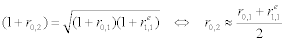Where rij denotes return of j-period bond at time i.

Consider an investor who decides how to invest fund over the next two periods. Interest rate on long-term bond equals the average of (expected) rates on short-term bonds over two periods. This relationship implies that monetary expansion is likely to reduce shortterm rates but increase long-term ones . Impulse response graphs show that money growth increases both short and long term rates. This can be explained by the fact that liquidity effect for short-term interest rate is relatively small (Figure 5) .

Cook and Hahn (1989) investigated monetary policy (changing FFRs target)’s impact on bond interest rate of diverse maturities. They found that money growth increases nominal interest rates at all horizons (Table 6).

2SLS Estimation
Summary of linear combination of coefficients
M1DIFF Lag(s) 0 to 2
Value          0.22
Standard error            0.07
t-statistic            2.99
Signif level            0.00

Table 6: Effects of money stock on output: Endogeneity.

We also replicate Cook and Hahn (1989)’s investigation substituting FFRs target for money growth. We found similar significant coefficient estimates at high levels of significance. This supports the original idea of them (Table 7).

Summary of linear combination of coefficients on;
M2DIFF           Lag(s) 0 to 12
Five nominal interest rates Federal funds rate
1-Month Certificates of deposit(CD)
1-Year Treasury bill
3-Year Treasury notes
10-Year Treasury notes
Value 1.65 t-statistic       7.13
Standard error 0.23 Signif level 0.00**
Value 1.45 t-statistic       5.93
Standard error 0.24 Signif level 0.00**
Value 1.52 t-statistic       7.62
Standard error 0.19 Signif level     0.00**
Value 1.47 t-statistic       7.85
Standard error 0.18 Signif level 0.00**
Value 1.42 t-statistic       s8.01
Standard error 0.17 Signif level 0.00**

Table 7: Impact of monetary changes on nominal interest rates (Term-Structure).

• Seventh, we can examine long-run equilibrium relationships between (real) money stock and output.

This supplements the previous literatures that focused on the shortterm relationships between variables.

If shocks are not correlated and have no contemporaneous interactions, the identification and interpretation of impulse response and forecast error variance are straightforward. At first, we performed tests for stationarity of series (Tables 8 and 9) [17-19].

 Regression Run From 1960:04 to 2000:04 Observations 162 With intercept with 6 lags on the differences Dickey-Fuller unit root test, Series REALM T-test statistic      -1.99806 Dickey-Fuller Unit root test, Series LOGGDP T-test statistic      -0.45701 Dickey-Fuller Unit root test, Series INFLA T-test statistic      -2.73634 Dickey-Fuller Unit root test, Series FFED T-test statistic      -2.71089 Dickey-Fuller Unit root test, Series FCM10 T-test statistic      -1.69772 Critical values: 1%=-3.463 5%=-2.875 10%=-2.574

Table 8: Tests for stationarity and cointegration.

Likelihood based analysis of cointegration
Unrestricted eigenvalues and -T log(1-lambda)
Rank EigVal Lambda-max Trace Trace-95% LogL
0         1608.2437
1 0.2629 49 121.2527 76 1632.9509
2 0.1583 27 71.8383 53 1646.9117
3 0.1337 23 43.9167 35 1658.5396
4 0.0779 13 20.6609 20 1665.1106
5 0.0454 7 7.5188 9 1668.8700

Table 9: Estimation of cointegration model.

The form of econometric model analyzing long-run relation is as follows. Estimated cointegrating vector (long-run relationship) is: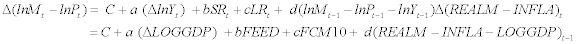Estimated error correction coefficient is significant, but does not ascertain stability (has positive sign) (Tables 10 and 11).

Coeff Std error T-stat Signif
EC1{1} 0.0016 0.000 3.036 0.002**

Table 10: Estimation of error-correction term.

Decomposition of variance for series REALM
Step Std error REALM LOGGDP INFLA FFED FCM10
1 0.00622146 100.000 0.000 0.000 0.000 0.000
2 0.01249448 87.860 2.657 0.182 6.484 2.816
35 0.12321185 9.607 11.636 10.764 6.414 1.578
36 0.12567639 69.555 11.275 11.048 6.577 1.545

Table 11: Decomposition of forecast error variance decomposition.

For VEC model, explanatory power for dependent variable (real money stock) is strongest in output, then next inflation.

#### Summary and Conclusion

In this study, we investigated the following issues.

• First, we reexamined St. Louis equation (output-money regression) incorporating sophisticated econometric issues.

Results seem to coincide with previous literatures, but we can find some new insights into monetary macroeconomic policy.

• Second, hypothesis tests for real vs. Keynesian fluctuation theories could be tested by advanced econometric methods. This can contribute to deriving appropriate policy implications from past data sets.

Our improvements can be summarized: smaller standard error in GLS, applying distributed lags and polynomial distributed lags models rather than OLS in St. Louis equation, deriving impulse response of GDP (output) to monetary shocks directly rather than the estimate for the sum of coefficients, finding structural break in M-Y relationships due to financial innovation, and replication the analyses for termstructure for changes in monetary policy.

We can approach more wide issues in future research. First, we can construct AD-AS model with structural VARs as Blanchard and Quah . They do not consider explicitly the effects on output of money growth. Second, we can modify money-output regression under fixed or floating exchange rates separately. Third, money-inflation relation is also important. We can decompose the effects of monetary policy into on output and the price level separately. Fourth, we can search natural experiments for determining the effects of monetary shocks. The death of the president of FRB of NY in 1928, brought monetary changes.

#### References

Select your language of interest to view the total content in your interested language

### Article Usage

• Total views: 12471
• [From(publication date):
August-2015 - Feb 19, 2020]
• Breakdown by view type
• HTML page views : 8590Can't read the image? click here to refresh